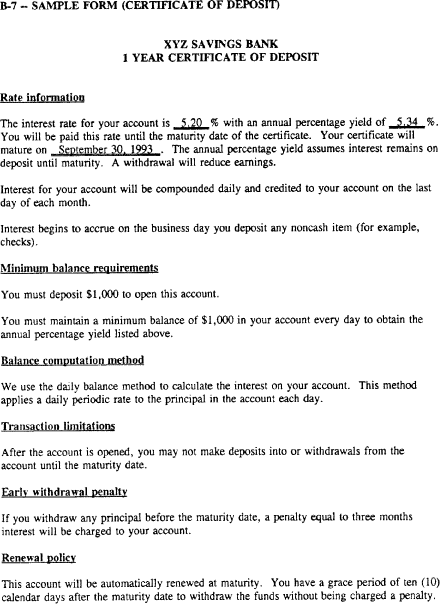An official website of the United States government

# Appendix B to Part 1030 — Model Clauses and Sample Forms

This version is the current regulation

1. Modifications. Institutions that modify the model clauses will be deemed in compliance as long as they do not delete required information or rearrange the format in a way that affects the substance or clarity of the disclosures.

2. Format. Institutions may use inserts to a document (see Sample Form B-4) or fill-in blanks (see Sample Forms B-5, B-6 and B-7, which use underlining to indicate terms that have been filled in) to show current rates, fees, or other terms.

3. Disclosures for opening accounts. The sample forms illustrate the information that must be provided to consumers when an account is opened, as required by § 1030.4(a)(1). (See § 1030.4(a)(2), which states the requirements for disclosing the annual percentage yield, the interest rate, and the maturity of a time account in responding to a consumer's request.)

4. Compliance with Regulation E. Institutions may satisfy certain requirements under Regulation DD with disclosures that meet the requirements of Regulation E. (See § 1030.3(c).) For disclosures covered by both this part and Regulation E (such as the amount of fees for ATM usage, institutions should consult appendix A to Regulation E for appropriate model clauses.

5. Duplicate disclosures. If a requirement such as a minimum balance applies to more than one account term (to obtain a bonus and determine the annual percentage yield, for example), institutions need not repeat the requirement for each term, as long as it is clear which terms the requirement applies to.

6. Sample forms. The sample forms (B-4 through B-8) serve a purpose different from the model clauses. They illustrate ways of adapting the model clauses to specific accounts. The clauses shown relate only to the specific transactions described.

### B-1 Model Clauses for Account Disclosures

B-1(h) Disclosures Relating to Time Accounts

1. Maturity. The disclosure in Clause (h)(i) stating a specific date may be used in all cases. The statement describing a time period is appropriate only when providing disclosures in response to a consumer's request.

1. General. The second clause, describing a future decrease in the interest rate and annual percentage yield, applies to fixed-rate accounts only.

### B-4 Sample Form (Multiple Accounts)

1. Rate sheet insert. In the rate sheet insert, the calculations of the annual percentage yield for the three-month and six-month certificates are based on 92 days and 181 days respectively. All calculations in the insert assume daily compounding.

### B-6 Sample Form (Tiered-Rate Money Market Account)

1. General. Sample Form B-6 uses Tiering Method A (discussed in appendix A and Clause (a)(iv)) to calculate interest. It gives a narrative description of a tiered-rate account; institutions may use different formats (for example, a chart similar to the one in Sample Form B-4), as long as all required information for each tier is clearly presented. The form does not contain a separate disclosure of the minimum balance required to obtain the annual percentage yield; the tiered-rate disclosure provides that information.

See interpretation of Appendix B in Supplement I

B-1 - Model Clauses for Account Disclosures (Section 1030.4(b))

B-2 - Model Clauses for Change in Terms (Section 1030.5(a))

B-3 - Model Clauses for Pre-Maturity Notices for Time Accounts (Section 1030.5(b)(2) and 1030.5(d))

B-4 - Sample Form (Multiple Accounts)

B-5 - Sample Form (Now Account)

B-6 - Sample Form (Tiered Rate Money Market Account)

B-7 - Sample Form (Certificate of Deposit)

B-10 - Sample Form (Aggregate Overdraft and Returned Item Fees)

## B-1 - Model Clauses for Account Disclosures

### (a) Rate Information

#### (i) Fixed-Rate Accounts

```The interest rate on your account is __% with an annual percentage yield of __%. You will be paid this rate [for (time period)/until (date)/for at least 30 calendar days].
```

#### (ii) Variable-Rate Accounts

```The interest rate on your account is __% with an annual percentage yield of __%.

Your interest rate and annual percentage yield may change.
```

#### Determination of Rate

```The interest rate on your account is based on (name of index) [plus/minus a margin of __]; or

At our discretion, we may change the interest rate on your account.
```

#### Frequency of Rate Changes

```We may change the interest rate on your account [every (time period)/at any time].
```

#### Limitations on Rate Changes

```The interest rate for your account will never change by more than __% each (time period).

The interest rate will never be [less/more] than __%; or

The interest rate will never [exceed__% above/drop more than __% below] the interest rate initially disclosed to you.
```

#### (iii) Stepped-Rate Accounts

```The initial interest rate for your account is __%. You will be paid this rate [for (time period)/until (date)]. After that time, the interest rate for your account will be __%, and you will be paid this rate [for (time period)/until (date)]. The annual percentage yield for your account is __%.
```

#### Tiering Method A

```• If your [daily balance/average daily balance] is \$__ or more, the interest rate paid on the entire balance in your account will be __% with an annual percentage yield of __%.

• If your [daily balance/average daily balance] is more than \$__, but less than \$__, the interest rate paid on the entire balance in your account will be __% with an annual percentage yield of __%.

• If your [daily balance/average daily balance] is \$__ or less, the interest rate paid on the entire balance will be __% with an annual percentage yield of __%.
```

#### Tiering Method B

```• An interest rate of __% will be paid only for that portion of your [daily balance/average daily balance] that is greater than \$__. The annual percentage yield for this tier will range from __% to __%, depending on the balance in the account.

• An interest rate of __% will be paid only for that portion of your [daily balance/average daily balance] that is greater than \$__. The annual percentage yield for this tier will range from __% to __%, depending on the balance in the account.

• If your [daily balance/average daily balance] is \$__ or less, the interest rate paid on the entire balance will be __% with an annual percentage yield of __%.
```

### (b) Compounding and Crediting

#### (i) Frequency

```Interest will be compounded [on a __ basis/every (time period)]. Interest will be credited to your account [on a __ basis/every (time period)].
```

#### (ii) Effect of Closing an Account

```If you close your account before interest is credited, you will not receive the accrued interest.
```

### (c) Minimum Balance Requirements

#### (i) To Open the Account

```You must deposit \$__ to open this account.
```

#### (ii) To Avoid Imposition of Fees

```A minimum balance fee of \$__ will be imposed every (time period) if the balance in the account falls below \$__ any day of the (time period).

A minimum balance fee of \$__ will be imposed every (time period) if the average daily balance for the (time period) falls below \$__. The average daily balance is calculated by adding the principal in the account for each day of the period and dividing that figure by the number of days in the period.
```

#### (iii) To Obtain the Annual Percentage Yield Disclosed

```You must maintain a minimum balance of \$__ in the account each day to obtain the disclosed annual percentage yield.

You must maintain a minimum average daily balance of \$__ to obtain the disclosed annual percentage yield. The average daily balance is calculated by adding the principal in the account for each day of the period and dividing that figure by the number of days in the period.
```

### (d) Balance Computation Method

#### (i) Daily Balance Method

```We use the daily balance method to calculate the interest on your account. This method applies a daily periodic rate to the principal in the account each day.
```

#### (ii) Average Daily Balance Method

```We use the average daily balance method to calculate interest on your account. This method applies a periodic rate to the average daily balance in the account for the period. The average daily balance is calculated by adding the principal in the account for each day of the period and dividing that figure by the number of days in the period.
```

### (e) Accrual of Interest on Noncash Deposits

```Interest begins to accrue no later than the business day we receive credit for the deposit of noncash items (for example, checks); or

Interest begins to accrue on the business day you deposit noncash items (for example, checks).
```

### (f) Fees

```The following fees may be assessed against your account:

__\$__

__\$__

__\$__

__(conditions for imposing fee) \$__

__% of __.
```

### (g) Transaction Limitations

```The minimum amount you may [withdraw/write a check for] is \$__.

You may make __ [deposits into/withdrawals from] your account each (time period).

You may not make [deposits into/withdrawals from] your account until the maturity date.
```

### (h) Disclosures Relating to Time Accounts

#### (i) Time Requirements

```Your account will mature on (date).

Your account will mature in (time period).
```

#### (ii) Early Withdrawal Penalties

```We [will/may] impose a penalty if you withdraw [any/all] of the [deposited funds/principal] before the maturity date. The fee imposed will equal __ days/week[s]/month[s] of interest; or

We [will/may] impose a penalty of \$__ if you withdraw [any/all] of the [deposited funds/principal] before the maturity date.

If you withdraw some of your funds before maturity, the interest rate for the remaining funds in your account will be __% with an annual percentage yield of __%.
```

#### (iii) Withdrawal of Interest Prior to Maturity

```The annual percentage yield assumes interest will remain on deposit until maturity. A withdrawal will reduce earnings.
```

#### (1) Automatically Renewable Time Accounts

```This account will automatically renew at maturity.

You will have [__ calendar/business] days after the maturity date to withdraw funds without penalty; or

There is no grace period following the maturity of this account to withdraw funds without penalty.
```

#### (2) Non-Automatically Renewable Time Accounts

```This account will not renew automatically at maturity. If you do not renew the account, your deposit will be placed in [an interest-bearing/a noninterest-bearing] account.
```

#### (v) Required Interest Distribution

```This account requires the distribution of interest and does not allow interest to remain in the account.
```

### (i) Bonuses

```You will [be paid/receive] [\$__/(description of item)] as a bonus [when you open the account/on (date) __].

You must maintain a minimum [daily balance/average daily balance] of \$__ to obtain the bonus.

To earn the bonus, [\$__/your entire principal] must remain on deposit [for (time period)/until (date)__].
```

## B-2 - Model Clauses for Change in Terms

```On (date), the cost of (type of fee) will increase to \$__.

On (date), the interest rate on your account will decrease to __% with an annual percentage yield of __%.

On (date), the minimum [daily balance/average daily balance] required to avoid imposition of a fee will increase to \$__.
```

## B-3 - Model Clauses for Pre-Maturity Notices for Time Accounts

### (a) Automatically Renewable Time Accounts With Maturities of One Year or Less But Longer Than One Month

```Your account will mature on (date).

If the account renews, the new maturity date will be (date).

The interest rate for the renewed account will be __% with an annual percentage yield of __%; or

The interest rate and annual percentage yield have not yet been determined. They will be available on (date). Please call (phone number) to learn the interest rate and annual percentage yield for your new account.
```

### (b) Non-Automatically Renewable Time Accounts With Maturities Longer Than One Year

```Your account will mature on (date).

If you do not renew the account, interest [will/will not] be paid after maturity.
```

## B-4—Sample Form (Multiple Accounts)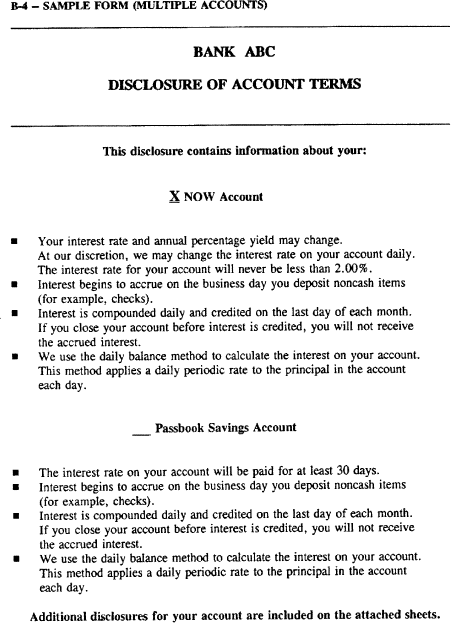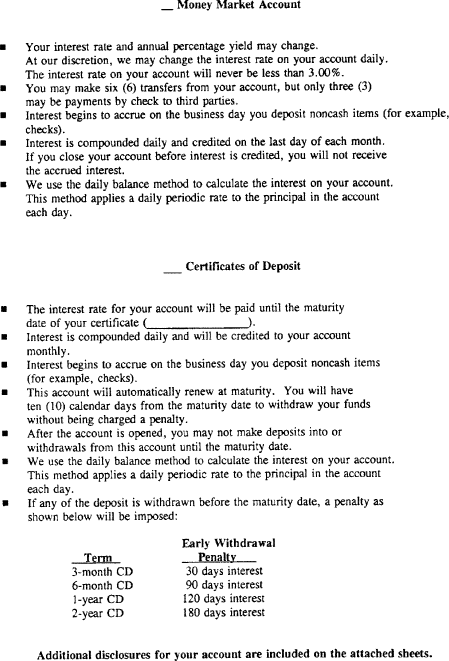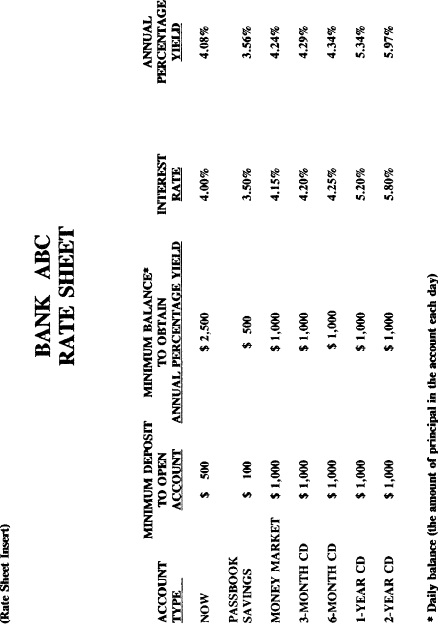## B-5—Sample Form (Now Account)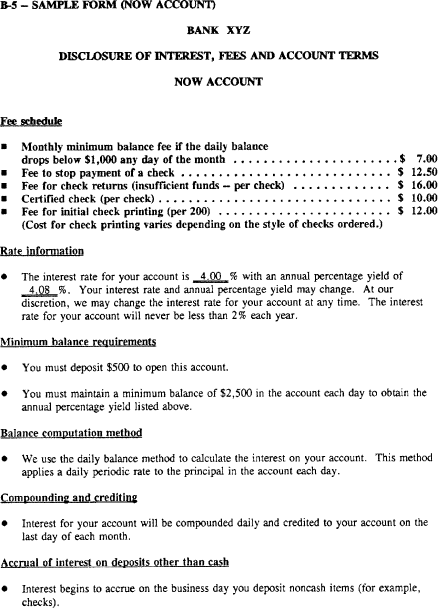## B-6—Sample Form (Tiered Rate Money Market Accounts)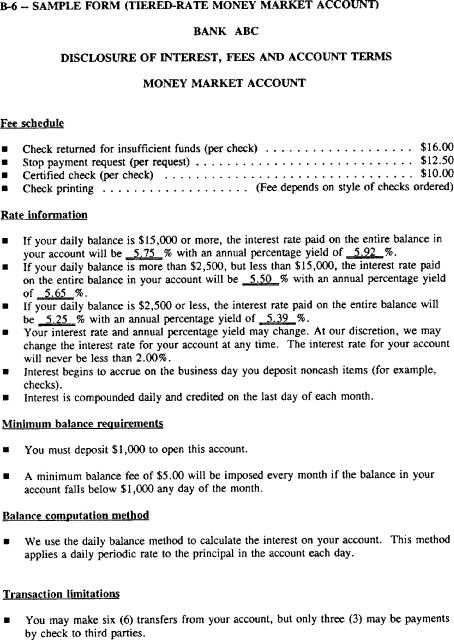## B-7—Sample Form (Certificate of Deposit)